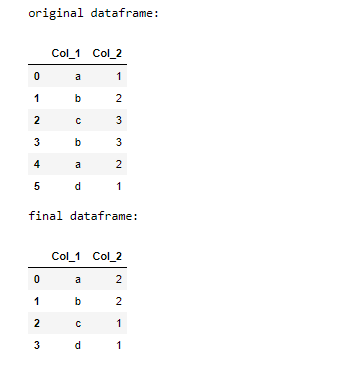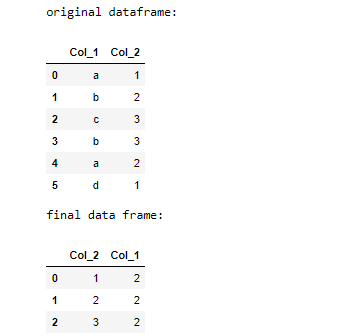Open In App
Related Articles
• Write an Interview Experience
• Pandas Exercises and Programs
• Different ways to create Pandas Dataframe

# How to count unique values in a Pandas Groupby object?

Here, we can count the unique values in Pandas groupby object using different methods. This article depicts how the count of unique values of some attribute in a data frame can be retrieved using Pandas.

### Method 1: Count unique values using nunique()

The Pandas dataframe.nunique() function returns a series with the specified axis’s total number of unique observations. The total number of distinct observations over the index axis is discovered if we set the value of the axis to 0.

## Python3

 `# import pandas``import` `pandas as pd` `# create dataframe``df ``=` `pd.DataFrame({``'Col_1'``: [``'a'``, ``'b'``, ``'c'``, ``'b'``, ``'a'``, ``'d'``],``                   ``'Col_2'``: [``1``, ``2``, ``3``, ``3``, ``2``, ``1``]})` `# call groupby method.``df ``=` `df.groupby(``'Col_1'``)[``'Col_2'``].nunique()` `display(df)`

Output:

```Col_1
a    2
b    2
c    1
d    1
Name: Col_2, dtype: int64```

### Method 2: Count unique values using agg()

Functions Used:

• The groupby() function is used to split the data into groups based on some criteria. Pandas’ objects can be split on any of their axes.
• The agg() is used to pass a function or list of functions to be applied on a series or even each element of a series separately. In the case of the list of functions, multiple results are returned by agg() method.
• Pandas reset_index() is a method to reset the index of a df.reset-index() method sets a list of integers ranging from 0 to the length of data as an index.

Example 1:

In the output, you will find that the elements present in col_1 counted the unique element present in that column, i.e, a is present 2 times.

## Python

 `# import pandas``import` `pandas as pd` `# create dataframe``df ``=` `pd.DataFrame({``'Col_1'``: [``'a'``, ``'b'``, ``'c'``, ``'b'``, ``'a'``, ``'d'``],``                   ``'Col_2'``: [``1``, ``2``, ``3``, ``3``, ``2``, ``1``]})` `# print original dataframe``print``(``"original dataframe:"``)``display(df)`  `# call groupby method.``df ``=` `df.groupby(``"Col_1"``)` `# call agg method``df ``=` `df.agg({``"Col_2"``: ``"nunique"``}).reset_index()` `# print dataframe``print``(``"final dataframe:"``)``display(df)`

Output:Example 2:

In the output, you will find that the elements present in col_2 counted the unique element present in that column, i.e,3 is present 2 times.

## Python

 `# import pandas``import` `pandas as pd` `# create dataframe``df ``=` `pd.DataFrame({``'Col_1'``: [``'a'``, ``'b'``, ``'c'``, ``'b'``, ``'a'``, ``'d'``],``                   ``'Col_2'``: [``1``, ``2``, ``3``, ``3``, ``2``, ``1``]})` `# print original dataframe``print``(``"original dataframe:"``)``display(df)`  `# call groupby method.``df ``=` `df.groupby(``"Col_2"``)` `# call agg method``df ``=` `df.agg({``"Col_1"``: ``"nunique"``}).reset_index()` `# print dataframe``print``(``"final data frame:"``)``display(df)`

Output: